﻿ Python: Sum all the items in a list - w3resource

# Python: Sum all the items in a list

## Python List: Exercise-1 with Solution

Write a Python program to sum all the items in a list.

Example - 1 :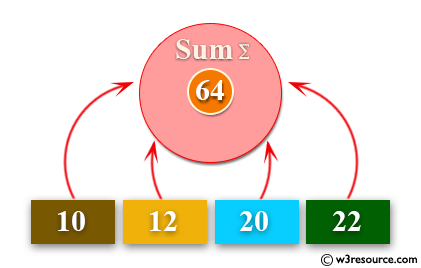Example - 2 :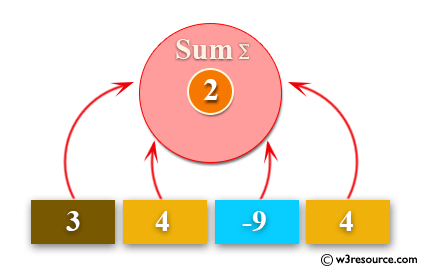Example - 3 :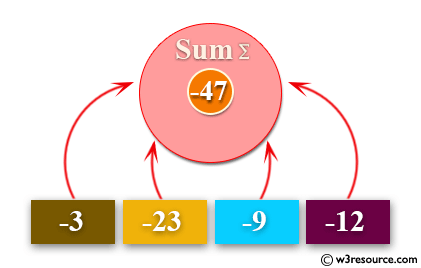Example - 4 :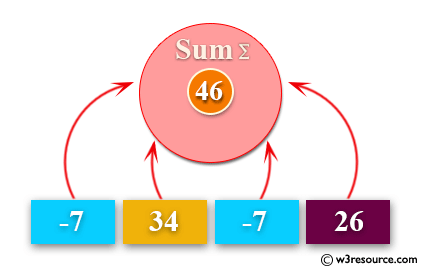Sample Solution:-

Python Code:

``````def sum_list(items):
sum_numbers = 0
for x in items:
sum_numbers += x
return sum_numbers
print(sum_list([1,2,-8]))
```
```

Sample Output:

```-5
```

Flowchart: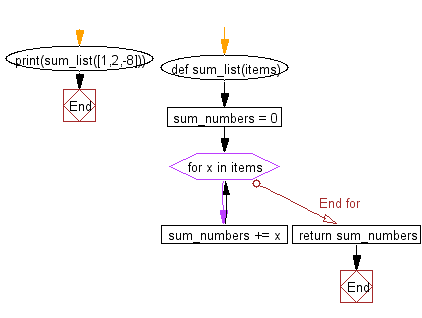## Visualize Python code execution:

The following tool visualize what the computer is doing step-by-step as it executes the said program:

Python Code Editor:

Have another way to solve this solution? Contribute your code (and comments) through Disqus.

What is the difficulty level of this exercise?

Test your Programming skills with w3resource's quiz.

﻿

## Python: Tips of the Day

Returns True if the provided function returns True for every element in the list, False otherwise:

Example:

```def tips_every(lst, fn=lambda x: x):
return all(map(fn, lst))

print(tips_every([2, 4, 3], lambda x: x > 1))
print(tips_every([1, 2, 3]))
```

Output:

```True
True
```